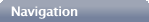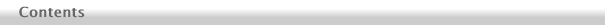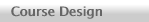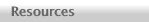The text chapters are listed below, or look at a PDF of the full table of contents or a PDF flow chart that shows how the sections of the book depend on each other.You can purchase a copy directly from the publisher here.New! Online Student Manual available here.Thomas A. Moore has been a professor in the Physics and Astronomy Department at Pomona College since 1987. He does theoretical research on detecting gravitational waves using LISA (now eLISA). Send him a message.Copyright © 2009, 2010, 2015 Thomas A. MooreThe Einstein Equation
20. The Stress-Energy Tensor
21. The Einstein Equation
22. Interpreting the Equation
23. The Schwarzschild Solution

Cosmology
24. The Universe Observed
25. A Metric for the Cosmos
26. Evolution of the Universe
27. Cosmic Implications
28. The Early Universe
29. CMB Fluctuations and Inflation

Gravitational Waves
30. Gauge Freedom
31. Detecting Gravitational Waves
32. Gravitational Wave Energy
33. Generating Gravitational Waves
34. Gravitational Wave Astronomy

Spinning Black Holes
35. Gravitomagnetism
36. The Kerr Metric
37. Kerr Particle Orbits
38. Ergoregion and Horizon
39. Negative-Energy Orbits

1. Introduction

Flat Spacetime
2. Review of Special Relativity
3. Four-Vectors
4. Index Notation

Tensors
5. Arbitrary Coordinates
6. Tensor Equations
7. Maxwell's Equations
8. Geodesics

Schwarzschild Black Holes
9. The Schwarzschild Metric
10. Particle Orbits
11. Precession of the Perihelion
12. Photon Orbits
13. Deflection of Light
14. Event Horizon
15. Alternative Coordinates
16. Black Hole Thermodynamics

The Calculus of Curvature